# Water detection with Sentinel-1¶

Keywords: data used; sentinel-1,:index:SAR, water

## Background¶

Over 40% of the world’s population lives within 100 km of the coastline. However, coastal environments are constantly changing, with erosion and coastal change presenting a major challenge to valuable coastal infrastructure and important ecological habitats. Updating data on the position of the coastline is essential for coastal managers to be able to identify and minimise the impacts of coastal change and erosion. The coastal regions are also home to many wetlands. Monitoring of water extent helps to understand and protect these dynamic and productive ecosystems.

While coastlines and water extents can be mapped using optical data (demonstrated in the Coastal Erosion notebook), these images can be strongly affected by the weather, especially through the presence of clouds, which obscure the land and water below. This can be a particular problem in cloudy regions or areas where clouds in wet season prevent optical satellites from taking clear images for many months of the year.

Radar observations are largely unaffected by cloud cover. The two Sentinel-1 satellites, operated by ESA as part of the Copernicus program, provide all-weather observations every 6 to 12 days over Africa. By developing a process to classify the observed pixels as either water or land, it is possible to identify the shoreline and map the dynamic water extents using radar data. For more information, see the Sentinel-1 notebook.

## Description¶

In this example, we use data from the Sentinel-1 satellites to build a classifier that can determine whether a pixel is a water or land. Specifically, this notebook uses analysis-ready radar backscatter, which describes the strength of the signal received by the satellite.

The notebook contains the following steps:

1. Load Sentinel-1 backscatter data for an area of interest and visualize the returned data

2. Applying speckle filter and converting the digital numbers to dB values for analysis

3. Use histogram analysis to determine the threshold for water classification

4. Design a classifier to distinguish land and water

5. Apply the classifier to the area of interest and interpret the results

## Getting started¶

To run this analysis, run all the cells in the notebook, starting with the “Load packages” cell.

Import Python packages that are used for the analysis.

:

%matplotlib inline

# Force GeoPandas to use Shapely instead of PyGEOS
# In a future release, GeoPandas will switch to using Shapely by default.
import os
os.environ['USE_PYGEOS'] = '0'

import warnings
import datacube
import numpy as np
import xarray as xr
import geopandas as gpd
import matplotlib.pyplot as plt
warnings.filterwarnings("ignore")

from scipy.ndimage.filters import uniform_filter
from scipy.ndimage.measurements import variance
from skimage.filters import threshold_minimum
from datacube.utils.geometry import Geometry

from deafrica_tools.spatial import xr_rasterize
from deafrica_tools.plotting import display_map, rgb
from deafrica_tools.areaofinterest import define_area


### Connect to the datacube¶

Connect to the datacube so we can access DEA data. The app parameter is a unique name for the analysis which is based on the notebook file name.

:

dc = datacube.Datacube(app="Radar_water_detection")


### Analysis parameters¶

The following cell sets the parameters, which define the area of interest and the length of time to conduct the analysis. The parameters are

• lat: The central latitude to analyse (e.g. 10.338).

• lon: The central longitude to analyse (e.g. -1.055).

• buffer: The number of square degrees to load around the central latitude and longitude. For reasonable loading times, set this as 0.1 or lower.

• time_range: The date range to analyse (e.g. ('2020'))

If running the notebook for the first time, keep the default settings below. This will demonstrate how the analysis works and provide meaningful results. The example covers the coast of Ziguinchor, Gambia.

### Select location¶

To define the area of interest, there are two methods available:

1. By specifying the latitude, longitude, and buffer. This method requires you to input the central latitude, central longitude, and the buffer value in square degrees around the center point you want to analyze. For example, lat = 10.338, lon = -1.055, and buffer = 0.1 will select an area with a radius of 0.1 square degrees around the point with coordinates (10.338, -1.055).

2. By uploading a polygon as a GeoJSON or Esri Shapefile. If you choose this option, you will need to upload the geojson or ESRI shapefile into the Sandbox using Upload Files button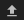in the top left corner of the Jupyter Notebook interface. ESRI shapefiles must be uploaded with all the related files (.cpg, .dbf, .shp, .shx). Once uploaded, you can use the shapefile or geojson to define the area of interest. Remember to update the code to call the file you have uploaded.

To use one of these methods, you can uncomment the relevant line of code and comment out the other one. To comment out a line, add the "#" symbol before the code you want to comment out. By default, the first option which defines the location using latitude, longitude, and buffer is being used.

The selected latitude and longitude will be displayed as a red box on the map below the next cell. This map can be used to find coordinates of other places, simply scroll and click on any point on the map to display the latitude and longitude of that location.

:

# Method 1: Specify the latitude, longitude, and buffer
aoi = define_area(lat=12.62, lon=-16.29, buffer=0.05)

# Method 2: Use a polygon as a GeoJSON or Esri Shapefile.
#aoi = define_area(vector_path='aoi.shp')

#Create a geopolygon and geodataframe of the area of interest
geopolygon = Geometry(aoi["features"]["geometry"], crs="epsg:4326")
geopolygon_gdf = gpd.GeoDataFrame(geometry=[geopolygon], crs=geopolygon.crs)

# Get the latitude and longitude range of the geopolygon
lat_range = (geopolygon_gdf.total_bounds, geopolygon_gdf.total_bounds)
lon_range = (geopolygon_gdf.total_bounds, geopolygon_gdf.total_bounds)

#timeframe
timerange = ('2020')


## View the selected location¶

The next cell will display the selected area on an interactive map. Feel free to zoom in and out to get a better understanding of the area you’ll be analysing. Clicking on any point of the map will reveal the latitude and longitude coordinates of that point.

:

display_map(x=lon_range, y=lat_range)

:

Make this Notebook Trusted to load map: File -> Trust Notebook

## Load and view Sentinel-1 data¶

The first step in the analysis is to load Sentinel-1 backscatter data for the specified area of interest. This uses the pre-defined load_ard utility function.

Please be patient. The data may take a few minutes to load and progress will be indicated by text output. The load is complete when the cell status goes from [*] to [number].

:

# Load the Sentinel-1 data
products=["s1_rtc"],
measurements=['vv', 'vh'],
y=lat_range,
x=lon_range,
time=timerange,
output_crs = "EPSG:6933",
resolution = (-20,20),
group_by="solar_day",
dtype='native'
)

Using pixel quality parameters for Sentinel 1
Finding datasets
s1_rtc


Once the load is complete, examine the data by printing it in the next cell. The Dimensions argument reveals the number of time steps in the data set, as well as the number of pixels in the x (longitude) and y (latitude) dimensions.

:

print(S1)

<xarray.Dataset>
Dimensions:      (time: 31, y: 623, x: 484)
Coordinates:
* time         (time) datetime64[ns] 2020-01-04T19:17:46.582116 ... 2020-12...
* y            (y) float64 1.604e+06 1.604e+06 ... 1.591e+06 1.591e+06
* x            (x) float64 -1.577e+06 -1.577e+06 ... -1.567e+06 -1.567e+06
spatial_ref  int32 6933
Data variables:
vv           (time, y, x) float32 0.05976 0.04359 0.02704 ... 0.1871 0.1521
vh           (time, y, x) float32 0.005369 0.004237 ... 0.01575 0.01733
Attributes:
crs:           EPSG:6933
grid_mapping:  spatial_ref


### Visualize the time series¶

Coastal and wetlands are highly dynamic, so cloud-free assessment using radar is valuable to capture change in all seasons. In the next few cells, Sentinel-1 observations in different polarisations are plotted for selected dates. Noting VV backscatter is usually significantly higher than VH. Signals from the two polarizations are dominated by different scattering mechanisms therefore respond differently to surface characeristics.

Both polarizations show siginficant surface cover change over time. Open water is characterized by low backscatter due to specular reflection. Water area with standing vegetation leads to high backscatter and will not be mapped using the thresholding method below. This may result in differenct water extent measurement compared to methods using optical data.

:

#Selecting a few images from the loaded S1 to visualise
timesteps = [2,4,6,9,11]

:

# Plot VV polarisation for specific timeframe
S1.vv.isel(time=timesteps).plot(cmap="Greys_r", robust=True, col="time", col_wrap=5);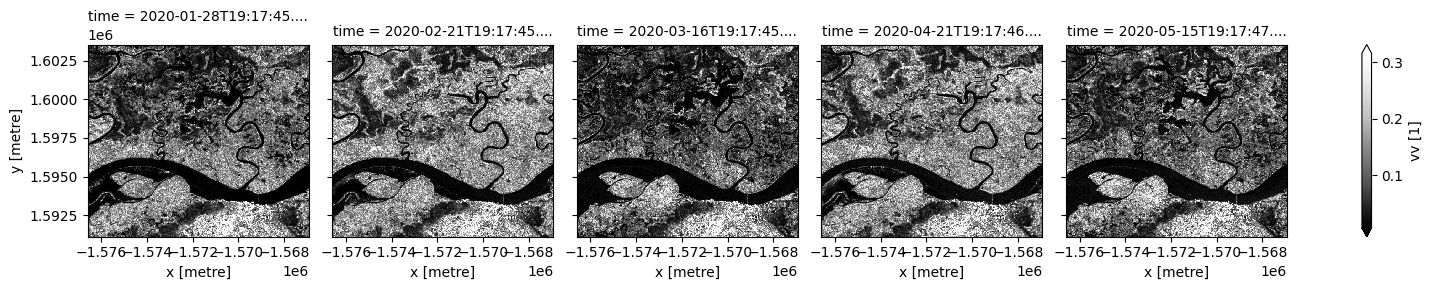:

# Plot VH polarisation for specific timeframe
S1.vh.isel(time=timesteps).plot(cmap="Greys_r", robust=True, col="time", col_wrap=5);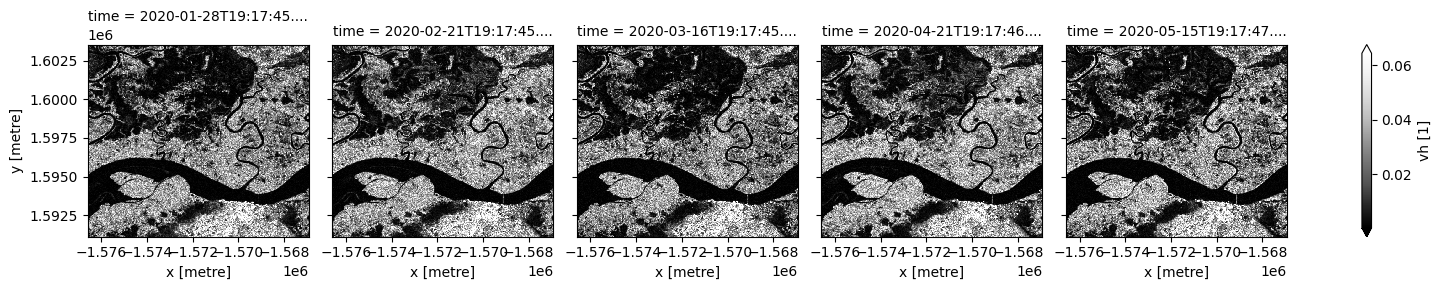Backscatter measurements can be combined in visualization to highlight the different polarization signatures. For the RGB visualization below, the ratio between VH and VV is added as a third measurement band.

:

# VH/VV is a potentially useful third feature after VV and VH
S1['vh/vv'] = S1.vh/S1.vv

:

# median values are used to scale the measurements so they have a similar range for visualization
med_s1 = S1[['vv','vh','vh/vv']].median()

:

# plotting an RGB image for selected timesteps
rgb(S1[['vv', 'vh', 'vh/vv']]/med_s1, bands=['vv',
'vh', 'vh/vv'], index=timesteps, col_wrap=5);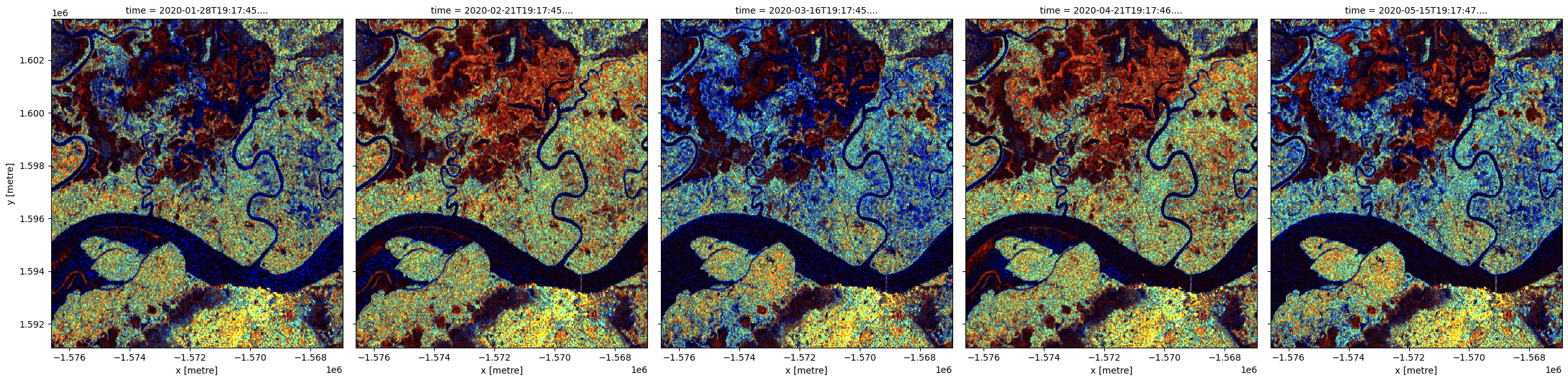Significant changes in color pattern are visible from month to month. Open water and smooth bare surface appear dark blue because all measurements are low. Tree cover appears cyan because of the relatively higher VH signal from volume scattering. Areas in bright red are likely water with standing vegetation. Urban area is shown in bright yellow at the bottom of the images.

## Apply speckle filtering¶

Radar observations appear speckly due to random interference of coherent signals from target scatters. The speckle noise can be reduced by averaging pixel values over an area or over time. However, averaging over a fixed window smoothes out real local spatial variation and leads to reduced spatial resolution. An adaptive approach that takes into account local homogeneity is therefore preferred.

Below, we apply the Lee filter, one of the popular adaptive speckle filters.

:

#defining a function to apply lee filtering on S1 image
def lee_filter(da, size):
"""
Apply lee filter of specified window size.

"""
img = da.values
img_mean = uniform_filter(img, (size, size))
img_sqr_mean = uniform_filter(img**2, (size, size))
img_variance = img_sqr_mean - img_mean**2

overall_variance = variance(img)

img_weights = img_variance / (img_variance + overall_variance)
img_output = img_mean + img_weights * (img - img_mean)

return img_output


Now that we’ve defined the filter, we can run it on the VV and VH data. You might have noticed that the function takes a size argument. This will change how blurred the image becomes after smoothing. We’ve picked a default value for this analysis, but you can experiement with this if you’re interested.

:

# The lee filter above doesn't handle null values
# We therefore set null values to 0 before applying the filter
valid = np.isfinite(S1)
S1 = S1.where(valid, 0)

# Create a new entry in dataset corresponding to filtered VV and VH data
S1["filtered_vv"] = S1.vv.groupby("time").apply(lee_filter, size=7)
S1["filtered_vh"] = S1.vh.groupby("time").apply(lee_filter, size=7)

# Null pixels should remain null
S1['filtered_vv'] = S1.filtered_vv.where(valid.vv)
S1['filtered_vh'] = S1.filtered_vh.where(valid.vh)

:

# images appear smoother after speckle filtering
fig, ax = plt.subplots(1, 2, figsize=(15,5))
S1["filtered_vv"].isel(time=3).plot(ax = ax,robust=True)
S1["filtered_vh"].isel(time=3).plot(ax = ax,robust=True);
ax.set_title('VV')
ax.set_title('VH')
plt.tight_layout();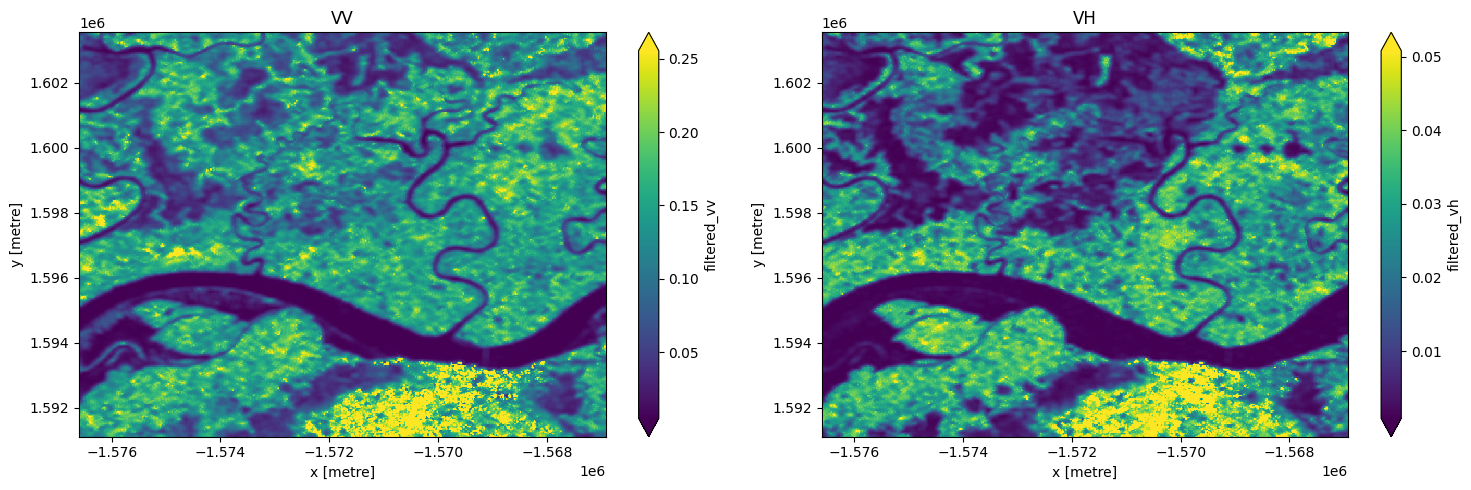### Convert the digital numbers to dB¶

While Sentinel-1 backscatter is provided as linear intensity, it is often useful to convert the backscatter to decible (dB) for analysis. Backscatter in dB unit has a more symmetric noise profile and less skewed value distribution for easier statistical evaluation.

:

S1['filtered_vv'] = 10 * np.log10(S1.filtered_vv)
S1['filtered_vh'] = 10 * np.log10(S1.filtered_vh)


## Histogram analysis for Sentinel-1¶

Backscatter distributions are plotted below as histograms.

:

fig = plt.figure(figsize=(12, 3))
S1.filtered_vh.plot.hist(bins=1000, label="VH filtered")
S1.filtered_vv.plot.hist(bins=1000, label="VV filtered",alpha=0.5)
plt.xlim(-40,-1)
plt.legend()
plt.xlabel("DN values in(dB)")
plt.title("Comparison of Lee filtered VH and VV polarisation bands");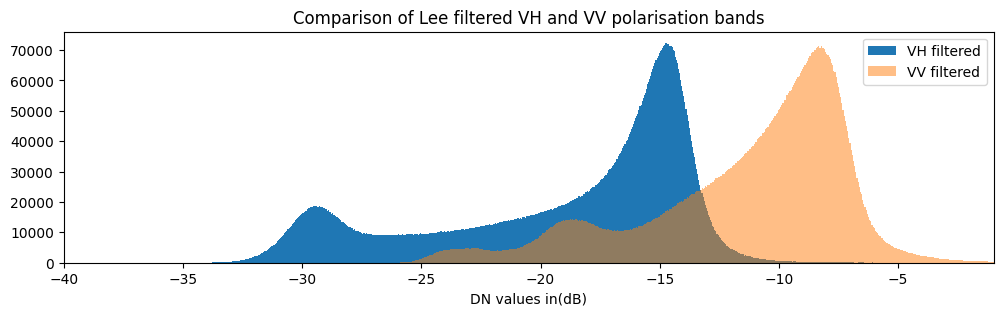## Build and apply the classifier¶

The histogram for VH backscatter shows a bimodal distribution with low values over water and high values over land. The VV histogram has multiple peaks and less obvious seperation between water and land.

We therefore build a classifier based on VH backscatter. We choose a threshold to separate land and water: pixels with values below the threshold are water, and pixels with values above the threshold are not water (land).

There are several ways to determine the threshold. Here, we use the threshod_minimum function implemented in the skimage package to determine the threshold from the VH histogram automatically. This method computes the histogram for all backscatter values, smooths it until there are only two maxima and find the minimum in between as the threshold.

:

threshold_vh = threshold_minimum(S1.filtered_vh.values)

print(threshold_vh)

-25.55215


### Visualise threshold¶

To check if our chosen threshold reasonably divides the two distributions, we can add the threshold to the histogram plots we made earlier.

:

fig, ax = plt.subplots(figsize=(15, 3))
S1.filtered_vh.plot.hist(bins=1000, label="VH filtered")
plt.xlim(-40,-5)
ax.axvspan(xmin=-40.0, xmax=threshold_vh, alpha=0.25, color="green", label="Water")
ax.axvspan(xmin=threshold_vh,
xmax=-5,
alpha=0.25,
color="red",
label="Not Water")
plt.legend()
plt.xlabel("VH (dB)")
plt.title("Effect of the classifier")
plt.show()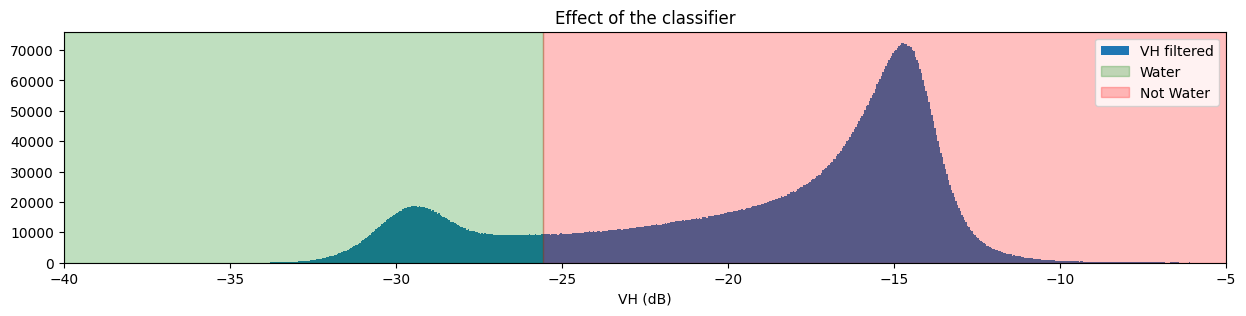### Define the classifier¶

This threshold is used to write a function to only return the pixels that are classified as water. The basic steps that the function will perform are:

1. Find all pixels that have filtered values lower than the threshold; these are the water pixels.

2. Return a data set containing the water pixels.

:

def S1_water_classifier(da, threshold=threshold_vh):
water_data_array = da < threshold
return water_data_array.to_dataset(name="s1_water")


Now that we have defined the classifier function, we can apply it to the data. After running the classifier, we will able to view the classified data product by running print(S1.water).

:

S1['water'] = S1_water_classifier(S1.filtered_vh).s1_water


### Assessment with mean¶

We can now view the image with our classification. The classifier returns either True or False for each pixel. To detect the boundaries of water features, we want to check which pixels are always water and which are always land. Conveniently, Python encodes True = 1 and False = 0.

If we plot the average classified pixel value, pixels that are always water will have an average value of 1 and pixels that are always land will have an average of 0. Pixels that are sometimes water and sometimes land will have an average between these values. In this case study, these pixels are associated with seasonally inundated wetland areas.

The following cell plots the average classified pixel value, or the frequency of water detection, over time.

:

# Plot the mean of each classified pixel value
plt.figure(figsize=(8, 7))
S1.water.mean(dim='time').plot(cmap="RdBu")
plt.title("Average classified pixel value");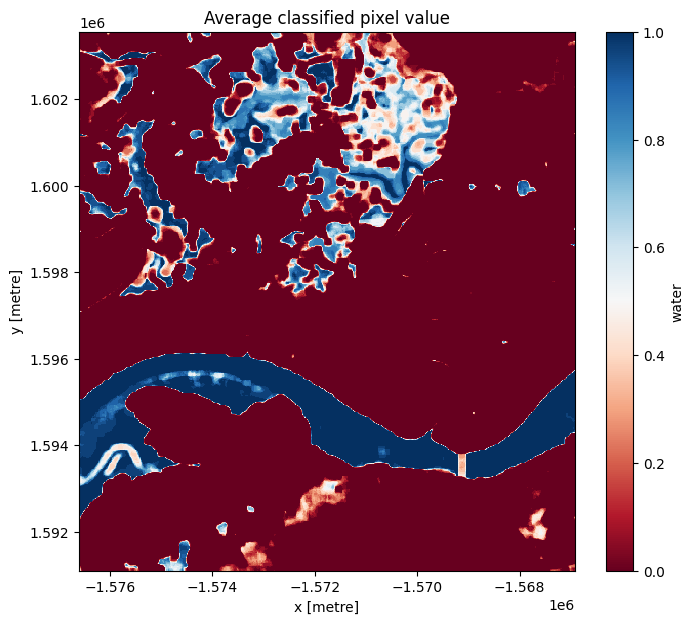You can see that the selected threshold has done a good job of separating the water pixels (in blue) and land pixels (in red) as well as ephemeral water features in between.

You should be able to see that the shoreline takes on a mix of values between 0 and 1, highlighting pixels that are sometimes land and sometimes water. This is likely due to the effect of rising and falling tides, with some radar observations being captured at low tide, and others at high tide.

### Assessment with standard deviation¶

Given that we’ve identified the shoreline as the pixels that are classified sometimes as land and sometimes as water, we can also see if the standard deviation of each pixel over time is a reasonable way to determine if a pixel is a shoreline or not.

Similar to how we calculated and plotted the mean above, you can calculate and plot the standard deviation by using the std function in place of the mean function.

If you’d like to see the results using a different colour scheme, you can also try substituting cmap="Greys" or cmap="Blues" in place of cmap="viridis".

:

plt.figure(figsize=(8, 7))
S1.water.std(dim="time").plot(cmap="viridis")
plt.title("Standard deviation of classified pixel values");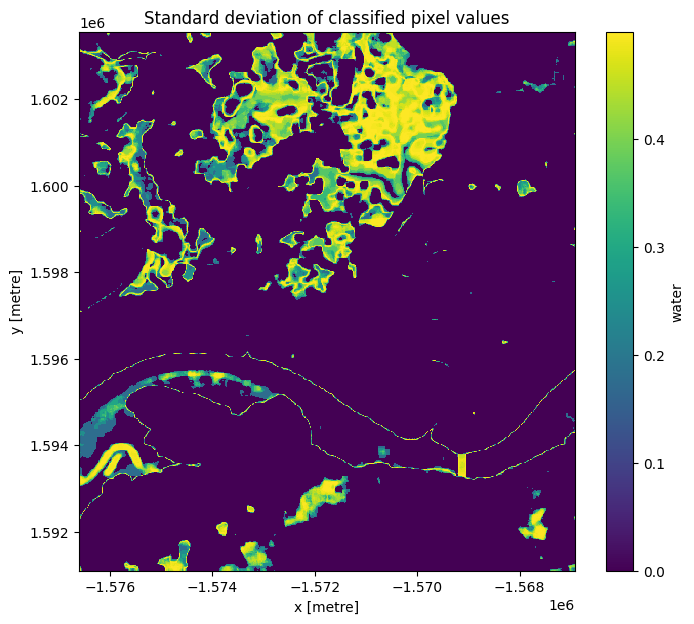The standard deviation we calculated above gives us an idea of how variable a pixel has been over the entire period of time that we looked at. From the image above, you should be able to see that the land and water pixels almost always have a standard deviation of 0, meaning they didn’t change over the time we sampled. The shoreline and wetlands however have a higher standard deviation, indicating that they change frequently between water and non-water.

An important thing to recognise is that the standard deviation might not be able to detect the difference between noise, tides, and ongoing change, since a pixel that frequently alternates between land and water (noise) could have the same standard deviation as a pixel that is land for some time, then becomes water for the remaining time (ongoing change or tides).

## Next steps¶

When you are done, return to the “Analysis parameters” section, modify some values (e.g. lat and lon) and rerun the analysis. You can use the interactive map in the “View the selected location” section to find new central latitude and longitude values by panning and zooming, and then clicking on the area you wish to extract location values for. You can also use Google maps to search for a location you know, then return the latitude and longitude values by clicking the map.

Contact: If you need assistance, please post a question on the Open Data Cube Slack channel or on the GIS Stack Exchange using the open-data-cube tag (you can view previously asked questions here). If you would like to report an issue with this notebook, you can file one on Github.

Compatible datacube version:

:

print(datacube.__version__)

1.8.15


Last Tested:

:

from datetime import datetime
datetime.today().strftime('%Y-%m-%d')

:

'2023-08-14'